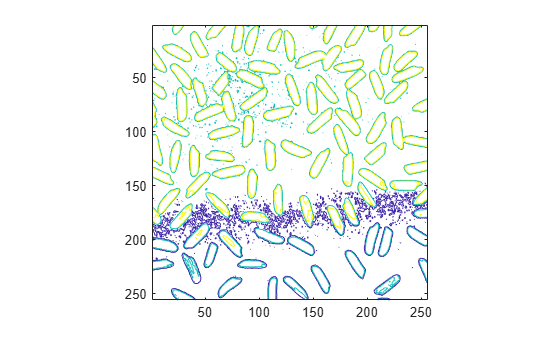# imcontour

Create contour plot of image data

## Syntax

``imcontour(I)``
``imcontour(I,levels)``
``imcontour(I,V)``
``imcontour(x,y,___)``
``imcontour(___,LineSpec)``
``[C,h] = imcontour(___)``

## Description

````imcontour(I)` draws a contour plot of the grayscale image `I`, choosing the number of levels and the values of levels automatically. `imcontour` automatically sets up the axes so their orientation and aspect ratio match the image.```

example

````imcontour(I,levels)` specifies the number, `levels`, of equally spaced contour levels in the plot.```
````imcontour(I,V)` draws contour lines at the data values specified in vector `V`. The number of contour levels is equal to `length(V)`.```
````imcontour(x,y,___)` uses the vectors `x` and `y` to specify the image x- and y coordinates.```
````imcontour(___,LineSpec)` draws the contours using the line type and color specified by `LineSpec`. Marker symbols are ignored.```
````[C,h] = imcontour(___)` returns the contour matrix, `C`, and the contour patches, `h`, that are drawn onto the current axes.```

## Examples

collapse all

Read and display a grayscale image.

```I = imread("rice.png"); imshow(I)```Create a contour plot of the image with three contour levels.

`imcontour(I,3);`## Input Arguments

collapse all

Grayscale image, specified as an m-by-n matrix.

Data Types: `single` | `double` | `int16` | `uint8` | `uint16` | `logical`

Number of contour levels, specified as a numeric scalar.

Value of contour levels, specified as a numeric vector with length greater than or equal to two. Use `V = [v v]` to compute a single contour at level `v`.

Image x values, specified as one of the following:

• 2-element numeric vector of the form ```[xmin xmax]``` — Image extent in the x direction.

• n-element numeric vector — x-coordinate of each column.

Image y values, specified as one of the following:

• 2-element numeric vector of the form ```[ymin ymax]``` — Image extent in the y direction.

• m-element numeric vector — y-coordinate of each row.

Line style and color, specified as a character vector or string scalar containing a line style specifier, a color specifier, or both.

Example: `"--r"` specifies red dashed lines

These two tables list the line style and color options.

Line StyleDescriptionResulting Line
`"-"`Solid line`"--"`Dashed line`":"`Dotted line`"-."`Dash-dotted lineColor SpecifierDescriptionAppearance

`r`

red`g`

green`b`

blue`c`

cyan`m`

magenta`k`

yellow`k`

black`w`

white## Output Arguments

collapse all

Contour matrix, returned as a matrix with two rows. The matrix is defined according to the `ContourMatrix` property of the `Contour` object, `h`.

Contour patches, returned as a `Contour` object.

## Version History

Introduced before R2006a